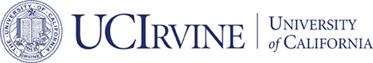# Submitted## Geometric Decompositions of Div-Conforming Finite Element Tensors

### Long Chen and Xuehai Huang

#### Submitted

ABSTRACT:

``` A unified construction of div-conforming finite element
tensors, including vector div element, symmetric div matrix element,
traceless div matrix element, and in general tensors with constraints,
is developed in this work. It is based on the geometric decomposition
of Lagrange elements into bubble functions on each sub-simplex. Then
the tensor at each sub-simplex is decomposed into the tangential and
the normal component. The tangential component forms the bubble
function space and the normal component characterizes the trace. A
deep exploration on boundary degrees of freedom is presented for
discovering various finite elements. The developed finite element
spaces are div conforming and satisfy the discrete inf-sup
condition. An explicit basis of the constraint tensor space is also
established.  ```